# ski-in / ski-out - Page 19

Viewing mode
697 results
•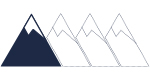• Slopes access :
• ski-in (-50 m)
• Rooms :
• Studio + cabin(s)
• Total surface (sq.m) :
• 30 sq.m
•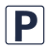•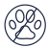•• Slopes access :
• 50 -300m to the slopes
• Rooms :
• Studio
• Studio + cabin(s)
• Total surface (sq.m) :
• 25 sq.m
•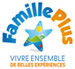•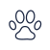••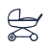• Slopes access :
• 50 -300m to the slopes
• Rooms :
• 5 rooms
• Total surface (sq.m) :
• 129 sq.m
••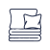•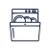•• Slopes access :
• 50 -300m to the slopes
• Rooms :
• 4 rooms
• 4 rooms + cabin(s)
• Total surface (sq.m) :
• 88 sq.m
••••• Slopes access :
• ski-in (-50 m)
• Rooms :
• Studio
• Studio + cabin(s)
• Total surface (sq.m) :
• 32 sq.m
•••• Slopes access :
• ski-in (-50 m)
• Rooms :
• Studio
• Studio + cabin(s)
• Total surface (sq.m) :
• 31 sq.m
••••• Slopes access :
• 50 -300m to the slopes
• Rooms :
• 3 rooms
• Total surface (sq.m) :
• 40 sq.m
•••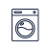••• Slopes access :
• 50 -300m to the slopes
• Rooms :
• Studio
• Studio + cabin(s)
• Total surface (sq.m) :
• 32 sq.m
•••• Slopes access :
• 50 -300m to the slopes
• Rooms :
• Studio
• Total surface (sq.m) :
• 16 sq.m
•• Slopes access :
• 50 -300m to the slopes
• Rooms :
• 2 rooms
• Total surface (sq.m) :
• 45 sq.m
•••••• Slopes access :
• ski-in (-50 m)
• Rooms :
• Studio
• Studio + cabin(s)
• Total surface (sq.m) :
• 34 sq.m
••• Slopes access :
• ski-in (-50 m)
• Rooms :
• Studio
• Studio + cabin(s)
• Total surface (sq.m) :
• 32 sq.m
•••• Slopes access :
• ski-in (-50 m)
• Rooms :
• 2 rooms
• 2 rooms + cabin(s)
• Total surface (sq.m) :
• 45 sq.m
•••• Slopes access :
• ski-in (-50 m)
• Rooms :
• 2 rooms
• Total surface (sq.m) :
• 40 sq.m
•••••• Slopes access :
• ski-in (-50 m)
• Rooms :
• 4 rooms
• 4 rooms + cabin(s)
•••••• Slopes access :
• 50 -300m to the slopes
• Rooms :
• 2 rooms
• 2 rooms + cabin(s)
• Total surface (sq.m) :
• 50 sq.m
•••••• Slopes access :
• ski-in (-50 m)
• Rooms :
• 5 rooms
• 5 rooms + cabin(s)
• Total surface (sq.m) :
• 115 sq.m
•••••• Slopes access :
• ski-in (-50 m)
• Rooms :
• 2 rooms
• 2 rooms + cabin(s)
• Total surface (sq.m) :
• 64 sq.m
••• Slopes access :
• 50 -300m to the slopes
• Rooms :
• Studio
• Studio + cabin(s)
• Total surface (sq.m) :
• 20 sq.m
••• Slopes access :
• ski-in (-50 m)
• Rooms :
• 3 rooms
• Total surface (sq.m) :
• 36 sq.m
•••